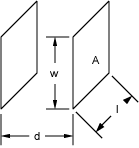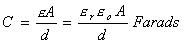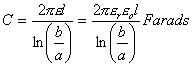NESS ENGINEERING
TECHNICAL DATA
CAPACITANCE FORMULAS

The formulas on this page allow one to calculate the capacitance for certain given geometries.  More complex problems typically require one to model the geometry and solve using Finite Element Analysis.

2 Parallel Plane Platese is the permittivity (F/m)  e0 is the permittivity of free space (F/m)  er is the relative permittivity of the dielectric  A is the area of the electrodes (length, l * width, w in m2)  d is the distance between the plates (m)

2 Coaxial Cylinderse is the permittivity (F/m)  e0 is the permittivity of free space (F/m)  er is the relative permittivity of the dielectric  a is the radius of the inner cylinder  b is the radius of the outer cylinder  l is the length of the cylinders (m)

Send consulting inquiries, comments, and suggestions to nessengr@san.rr.com

Ness Engineering, Inc.
P.O. Box 261501
San Diego, CA 92196
(858) 566-2372
(858) 240-2299 FAX

 © Richard M. Ness and Ness Engineering, Inc. Last Updated: